# Methods of measurements of Correlation

### What is Correlation?

A statistical tool that helps in the study of the relationship between two variables is known as Correlation. It also helps in understanding the economic behaviour of the variables. However, correlation does not tell anything about the cause-and-effect relationship between the two variables. Correlation can be measured through three different methods; viz., Scatter Diagram, Karl Pearson’s Coefficient of Correlation, and Spearman’s Rank Correlation Coefficient.

According to L.R. Connor, “If two or more quantities vary in sympathy so that movements in one tend to be accompanied by corresponding movements in others, then they are said to be correlated.”

### Methods of Measurements of Correlation

The three different methods of measuring correlation between two variables are:

1. Scatter Diagram
2. Karl Pearson’s Coefficient of Correlation
3. Spearman’s Rank Correlation Coefficient

### 1. Scatter Diagram:

A simple and attractive method of measuring correlation by diagrammatically representing bivariate distribution for determination of the nature of the correlation between the variables is known as Scatter Diagram Method. This method gives a visual idea to the investigator/analyst regarding the nature of the association between the two variables. It is the simplest method of studying the relationship between two variables as there is no need to calculate any numerical value.

#### How to draw a Scatter Diagram?

The two steps required to draw a Scatter Diagram or Dot Diagram are as follows:

1. Plot the values of the given variables (say X and Y) along the X-axis and Y-axis respectively.
2. Show these plotted values on the graph by dots. Each of these dots represents a pair of values.

#### Example:

Represent the following values of X and Y variables with the help of a scatter diagram. Also, comment on the type and degree of correlation.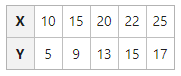#### Solution: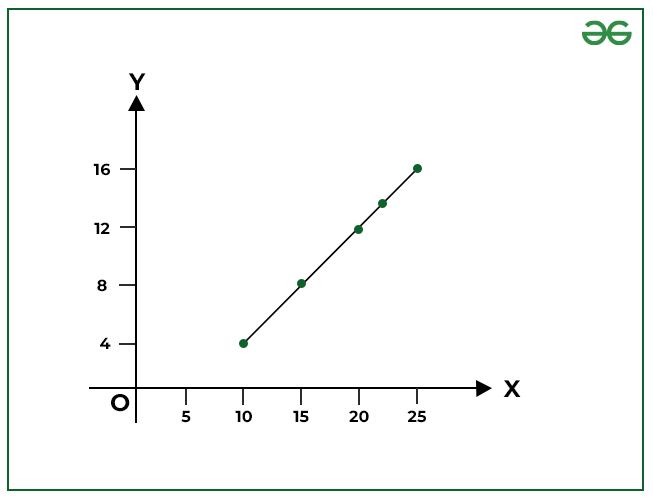The scatter diagram shows that there is an upward trend of the points from the lower left-hand corner to the upper right-hand corner of the graph. In short, there is a Positive Correlation between the values of X and Y variables.

### 2. Karl Pearson’s Coefficient of Correlation:

The first person to give a mathematical formula for the measurement of the degree of relationship between two variables in 1890 was Karl Pearson. Karl Pearson’s Coefficient of Correlation is also known as Product Moment Correlation or Simple Correlation Coefficient. This method of measuring the coefficient of correlation is the most popular and is widely used. It is denoted by ‘r’, where r is a pure number which means that r has no unit.

According to Karl Pearson, “Coefficient of Correlation is calculated by dividing the sum of products of deviations from their respective means by their number of pairs and their standard deviations.”OrWhere,

N = Number of Pair of Observations

x = Deviation of X series from Meany = Deviation of Y series from Mean= Standard Deviation of X series= Standard Deviation of X series= Standard Deviation of Y series= Standard Deviation of Y seriesr = Coefficient of Correlation

#### Example:

Use Actual Mean Method and determine Karl Pearson’s coefficient of correlation for the following data: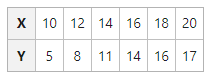#### Solution: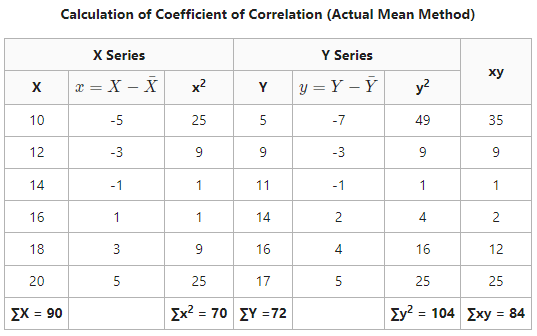∑xy = 84, ∑x2 = 70, ∑y2 = 104Coefficient of Correlation = 0.98

It means that there is a positive correlation between the values of Series X and Series Y.

### 3. Spearman’s Rank Correlation Coefficient:

Spearman’s Rank Correlation Coefficient or Spearman’s Rank Difference Method or Formula is a method of calculating the correlation coefficient of qualitative variables and was developed in 1904 by Charles Edward Spearman. In other words, the formula determines the correlation coefficient of variables like beauty, ability, honesty, etc., whose quantitative measurement is not possible. Therefore, these attributes are ranked or put in the order of their preference.In the given formula,

rk = Coefficient of rank correlation

D = Rank differences

N = Number of variables

Example:

Calculate Spearman’s Rank Correlation of Coefficient from the ranks given below: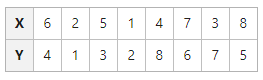Solution: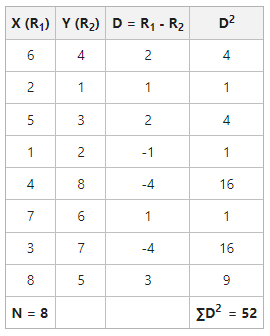= 1 – 0.619

= 0.38

Coefficient of Correlation (rk) = 0.38

As the rank correlation is positive and closer to 0, it means that the association between the ranks of X and Y is weaker.